﻿ 舰船大容量蓄电池组短路尖端放电故障机理研究
 舰船科学技术2020, Vol. 42Issue (1): 128-131PDF

Research on tip discharge mechanism in short-circuit process of large capacity battery for ship
XU Zheng-xi, CAI Jiu-qing, ZHENG Zhong-xiang, CHEN Tao
Wuhan Second Ship Design and Research Institute, Wuhan 430064, China
Abstract: As the most important backup power source and emergency power supply in ship, the operational safety of the battery is directly related to the combat effectiveness and safety of the ship. This paper focuses on the failure phenomenon of the battery pack caused by the short circuit of voltage detection lines. The modal analysis of the short circuit process is carried out based on the established short-circuit fault model. Then, the generation and transmission path of short circuit energy is expounded. The air gap breakdown and tip discharge phenomenon of the short circuit fault is explained by physical mechanism. Finally, based on the key source of short-circuit fault, the multilevel design method with system level, structure level, protection level and insulation level are proposed.
Key words: battery     short circuit     peak voltage     gap breakdown     tip discharge
0 引　言

1 系统结构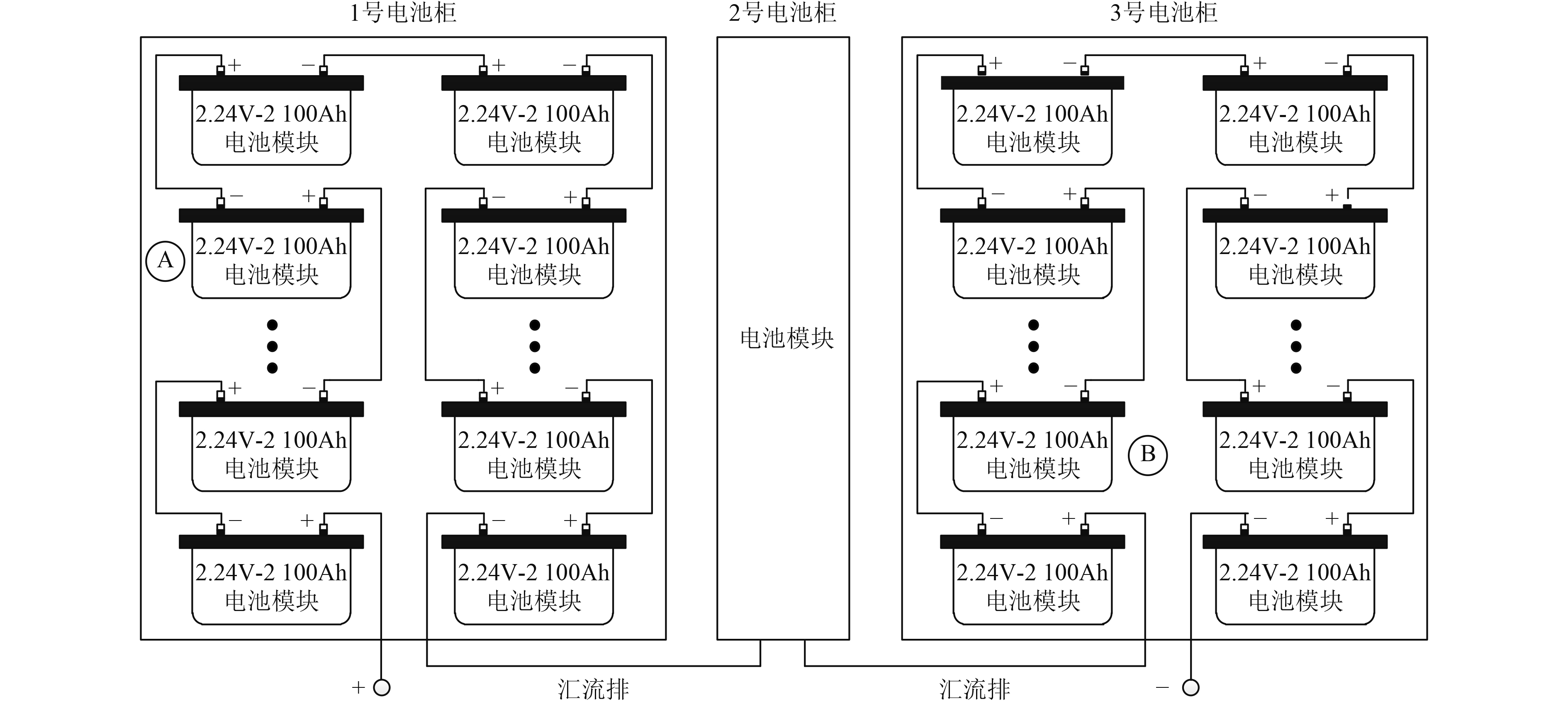图 1 大容量蓄电池组系统组成 Fig. 1 Composition of large capacity battery system
2 蓄电池组短路故障暂态分析 2.1 短路故障现象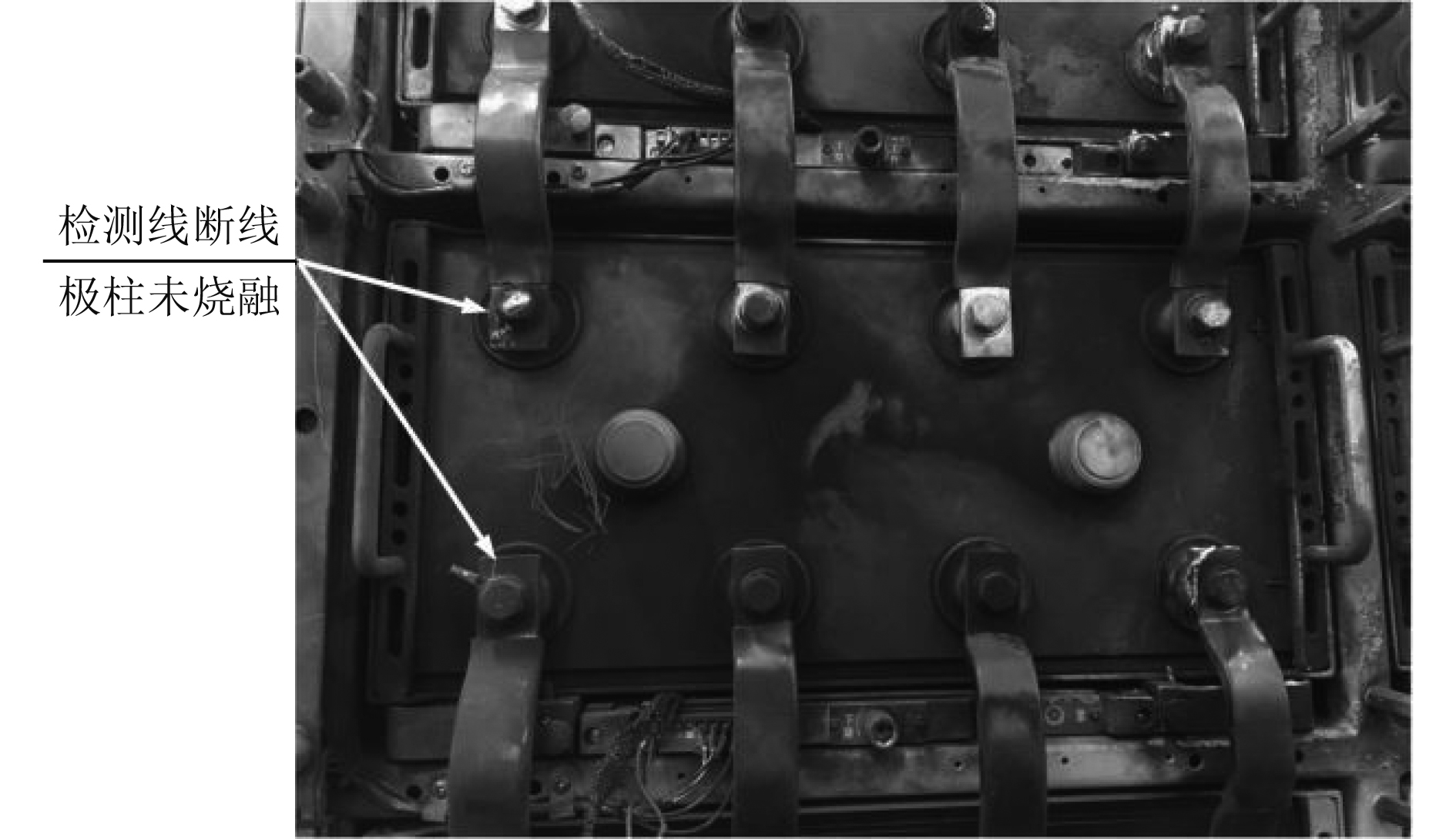图 2 第A节电池模块烧损部位图 Fig. 2 Burning region of A battery module.

B节电池模块极柱连接至接线端子排的一根电压检测线熔断，同时其附近数节电池模块及电池柜体也均有灼烧变黑痕迹。与第A节电池模块不同的是，第B节电池模块极柱及连接极柱的铜排不仅有电弧灼烧痕迹，同时伴有明显的烧融痕迹，故障部位及现象如图3所示。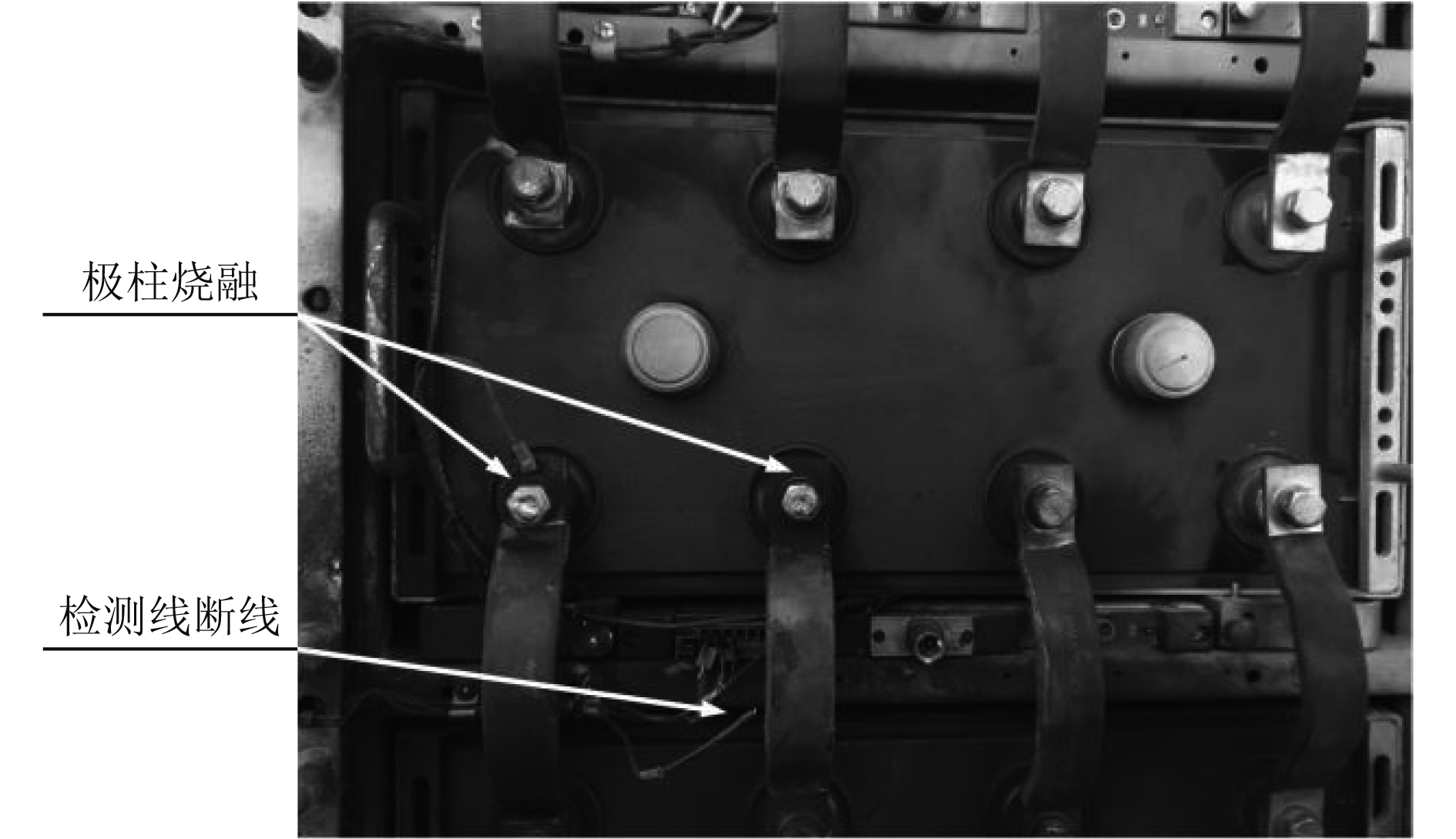图 3 第B节电池模块烧损部位图 Fig. 3 Burning region of B battery module.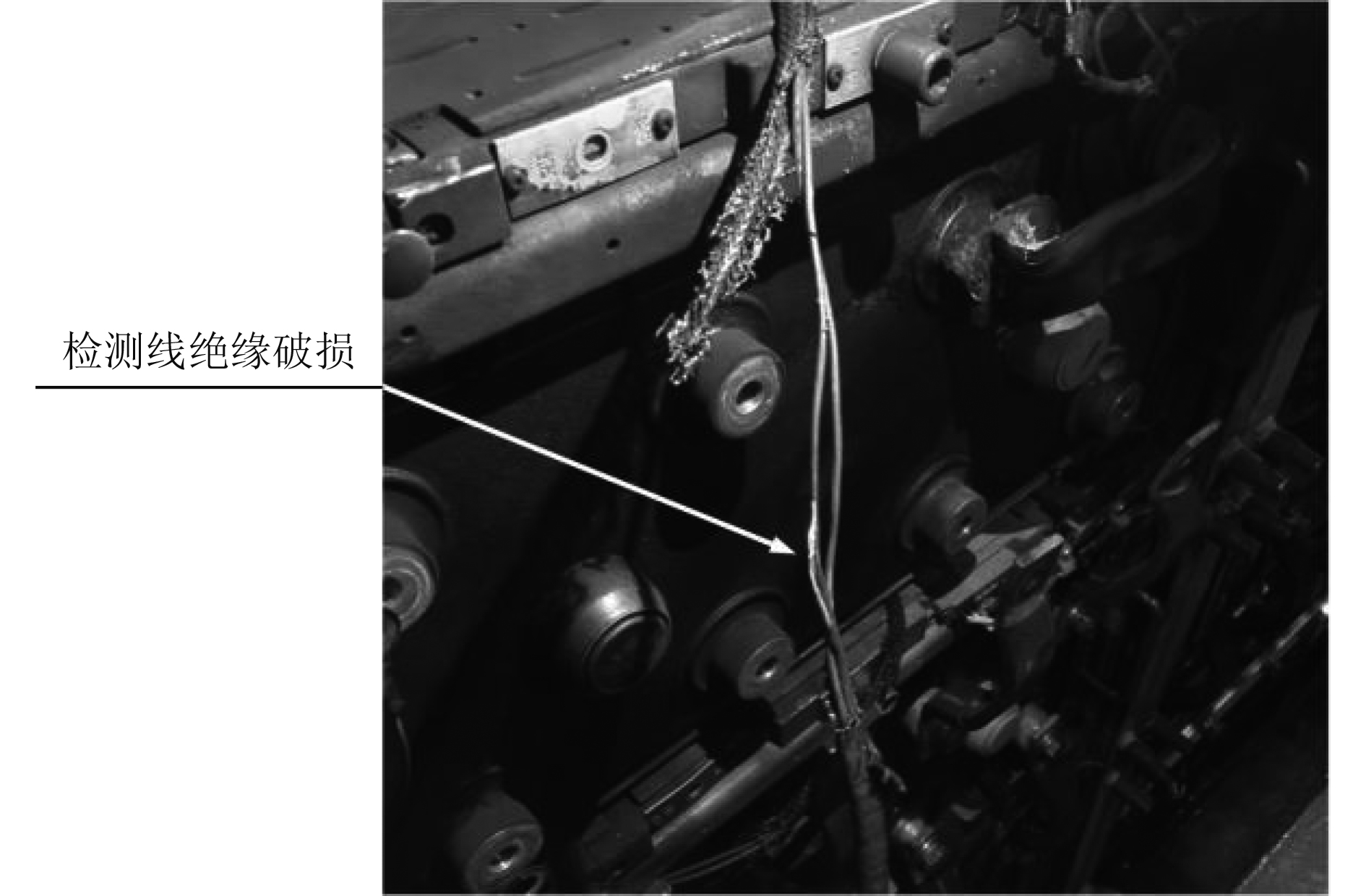图 4 电池模块电压检测线绝缘皮破损部位图 Fig. 4 Insulation damage region of voltage detection lines.
2.2 短路故障数学建模与模态分析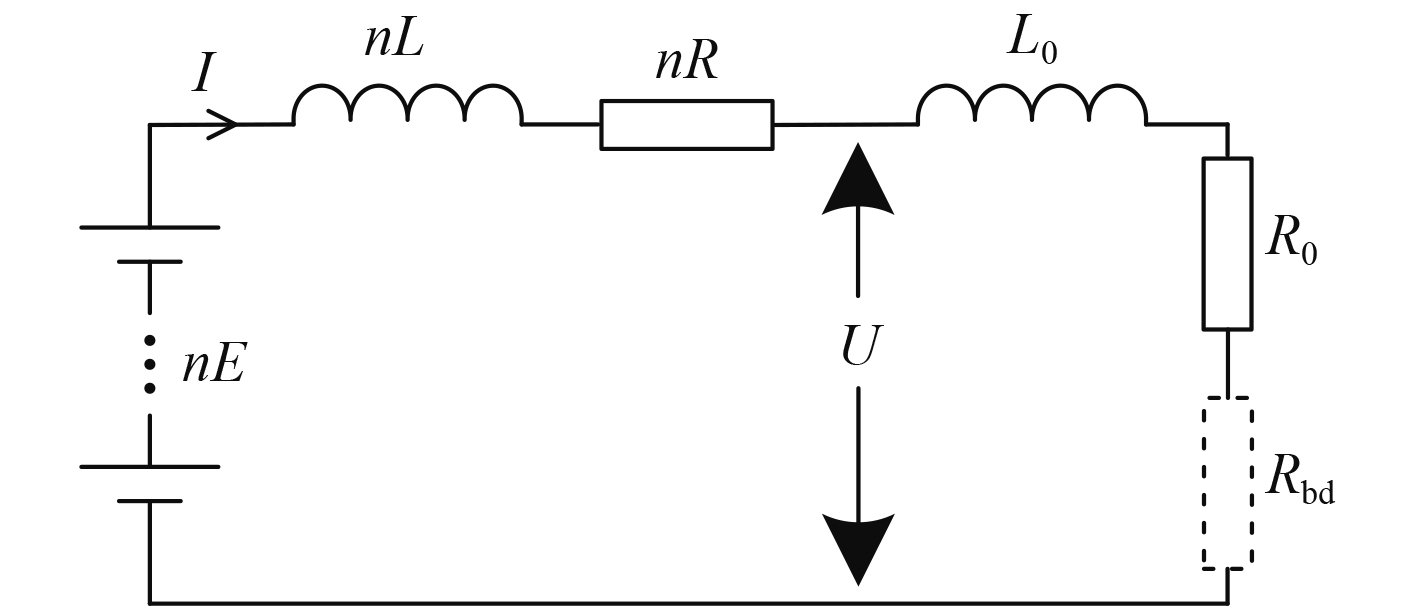图 5 蓄电池组短路故障等效电路 Fig. 5 Equivalent circuit of short-circuit fault with battery system.

1）短路电流上升阶段

 $nE = (nR + {R_0})I + (nL + {L_0})\frac{{{\rm d}I}}{{{\rm d}t}},\;I(0) = 0\text{。}$ (1)

2）第A节电池模块电压检测线熔断阶段

 $Q = cm\Delta T{\rm{ = }}\int_0^{{t_0}} {{I^2}{R_{0{\rm{A}}}}}{\rm d}t\text{，}$ (2)
 ${R_{0{\rm{A}}}} = {R_{0\_{{20}^ \circ }{\rm{C}}}}{\rm{ + }}{\alpha _{\rm{R}}} \cdot \Delta T \cdot {R_{0\_{{20}^ \circ }{\rm{C}}}}\text{。}$ (3)

3）第A节电池模块气隙击穿阶段

A节电池模块电压检测线熔断后，短路电流急剧下降，此时电流变化率dI/dt很大，在蓄电池组端口形成很高的尖峰电压。当尖峰电压值超过电池模块极柱与电池柜体间气隙击穿电压时，第A节电池模块极柱对电池柜体尖端放电。该阶段动态过程可以由下列方程式描述：

 ${U_{{t_1}}} = nE{\rm{ - }}nRI{\rm{ - }}nL\frac{{{\rm d}I}}{{{\rm d}t}},\;I(0) = {I_{{t_0}}},\;I({t_1}) = 0\text{，}$ (4)
 ${U_{{t_1}}} > {U_{bd}} = {E_{bd}} \cdot x\text{。}$ (5)

4）第B节电池模块电压检测线熔断阶段

A节电池模块极柱对电池柜体尖端放电，使得短路回路重新形成，由于在第A节电池模块电压检测线熔断阶段，急剧上升的电流同样在第B节电池模块电压检测线上积累产生了大量热量，因此此阶段持续时间很短，即会使第B节电池模块的电压检测线熔断，熔断过程短路电流同样急剧上升。该阶段动态过程可以由下列方程式描述：

 $nE = (nR + {R_0}{\rm{ + }}{R_{bd}})I + (nL + {L_0})\frac{{{\rm d}I}}{{{\rm d}t}},\;I(0) = 0\text{，}$ (6)
 $Q = cm\Delta T{\rm{ = }}\int_0^{{t_2}} {{I^2}{R_{0{\rm{B}}}}} {\rm d}t\text{。}$ (7)

5）第B节电池模块气隙击穿阶段

 ${U_{{t_3}}} = nE{\rm{ - }}nRI{\rm{ - }}nL\frac{{{\rm d}I}}{{{\rm d}t}},\;I(0) = {I_{{t_2}}},\;I({t_3}) = 0\text{，}$ (8)
 ${U_{{t_3}}} > {U_{bd}} = {E_{bd}} \cdot x\text{。}$ (9)

6）熄弧阶段

 ${Q_{{t_3}}}{\rm{ - }}\int_{{t_3}}^{{t_4}} {{I^2}(nR + {R_0}{\rm{ + 2}}{R_{bd}})} {\rm d}t < {Q_{_{bd}}}\text{。}$ (10)
2.3 短路故障现象物理机理

3 数值分析与计算

4 结　语

  凌虎. 蓄电池均衡充电与管理系统研究[D]. 合肥: 合肥工业大学馆, 2012. LING Hu. Research on equalizing charging and managing system for series-connected batteries[J]. Hefei University of Technology, 2012. http://cdmd.cnki.com.cn/Article/CDMD-10359-1012520861.htm  徐国顺, 庄劲武, 杨锋, 等. 大容量蓄电池组的数学建模及短路特性研究[J]. 低压电器, 2006, 11: 18-21. XU Guoshun, ZHUANG Jingwu, YANG Feng, et al. Research on mathematic model and short-circuit characteristics of high-capacity storage battery[J]. Low Voltage Apparatus, 2006, 11: 18-21.  申远, 尹静, 陈晓婷, 等. 蓄电池短路保护电路设计及应用研究[J]. 安徽大学学报, 2014, 38(3): 56-60. SHEN Yuan, YIN Jing, CHEN Xiaoting, et al. Design and applied research of short-circuit protection circuit for battery packs[J]. Journal of Anhui University, 2014, 38(3): 56-60. DOI:10.3969/j.issn.1000-2162.2014.03.010  钟久明, 刘树林, 王玉婷, 等. 短间隙的击穿及其短路放电特性研究[J]. 电工电能新技术, 2014, 33(1): 357-360. ZHONG Jiuming, LIU Shulin, WANG Yuting, et al. Study on breakdown and short circuit character of short gaps[J]. Advanced Technology of Electrical Engineering and Energy, 2014, 33(1): 357-360.  杨涛, 王露, 陈楠, 等. 直流局部放电干扰源研究[J]. 高压电器, 2012, 48(9): 72-2390. YANG Tao, WANG Lu, CHEN Nan, et al. Investigation in DC partial discharge interference sources[J]. High Voltage Apparatus, 2012, 48(9): 72-2390.